# Maharashtra Board Class 10 Maths Solutions Chapter 6 Trigonometry Problem Set 6

## Maharashtra State Board Class 10 Maths Solutions Chapter 6 Trigonometry Problem Set 6

Question 1.
Choose the correct alternative answer for the following questions.

i. sin θ.cosec θ = ?
(A) 1
(B) 0
(C) $$\frac { 1 }{ 2 }$$
(D) $$\sqrt { 2 }$$
(A)

ii. cosec 45° = ?
(A) $$\frac{1}{\sqrt{2}}$$
(B) $$\sqrt { 2 }$$
(C) $$\frac{\sqrt{3}}{2}$$
(D) $$\frac{1}{\sqrt{3}}$$
(B)

iii. 1 + tan2 θ = ?
(A) cot2 θ
(B) cosec2 θ
(C) sec2 θ
(D) tan2 θ
(C)

iv. When we see at a higher level, from the horizontal line, angle formed is ______
(A) angle of elevation.
(B) angle of depression.
(C) 0
(D) straight angle.
(A)

Question 2.
If sin θ = $$\frac { 11 }{ 61 }$$, find the value of cos θ using trigonometric identity.
Solution:
sin θ = $$\frac { 11 }{ 61 }$$ … [Given]
We know that,
sin2 θ + cos2 θ = 1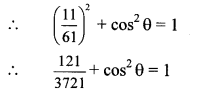…[Taking square root of both sides]

Question 3.
If tan θ = 2, find the values of other trigonometric ratios.
Solution:
tan θ = 2 …[Given]
We know that,
1 + tan2 θ = sec7 θ
∴ 1 + (2)7 = sec7 θ
∴ 1 + 4 = sec7 θ
∴ sec7 θ = 5
∴ sec θ = $$\sqrt { 5 }$$ …[Taking square root of both sides]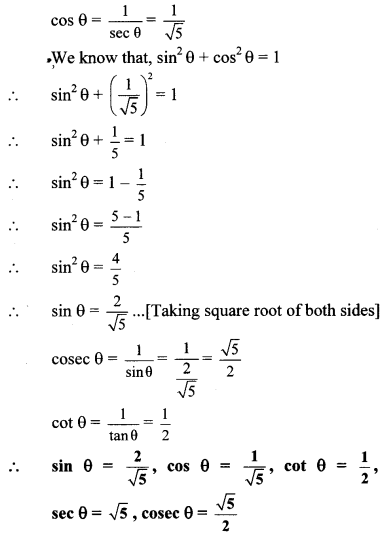Question 4.
If sec θ = $$\frac { 13 }{ 12 }$$, find the values of other trigonometric ratios.
Solution:
sec θ = $$\frac { 13 }{ 12 }$$ … [Given]
We know that,
1 + tan2 θ = sec2 θ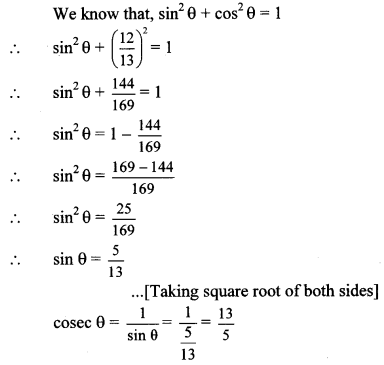∴ sin θ = $$\frac { 5 }{ 13 }$$, cos θ = $$\frac { 12 }{ 13 }$$, tan θ = $$\frac { 5 }{ 12 }$$, cot θ = $$\frac { 12 }{ 5 }$$, cosec θ = $$\frac { 13 }{ 5 }$$

Question 5.
Prove the following:
i. sec θ (1 – sin θ) (sec θ + tan θ) = 1
ii. (sec θ + tan θ) (1 – sin θ) = cos θ
iii. sec2 θ + cosec2 θ = sec2 θ × cosec2 θ
iv. cot2 θ – tan2 θ = cosec2 θ – sec2 θ
v. tan4 θ + tan2 θ = sec4 θ – sec2 θ
vi. $$\frac{1}{1-\sin \theta}+\frac{1}{1+\sin \theta}$$ = 2 sec2 θ
vii. sec6 x – tan6 x = 1 + 3 sec2 x × tan2 xProof:
i. L.H.S. = sec θ (1 – sin θ) (sec θ + tan θ)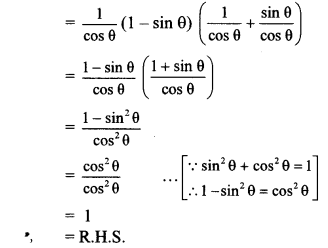∴ sec θ (1 – sin θ) (sec θ + tan θ) = 1

ii. L.H.S. = (sec θ + tan θ) (1 – sin θ)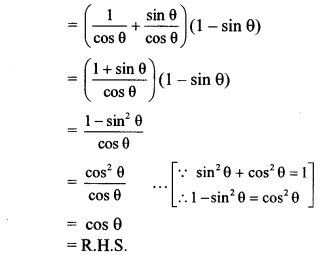∴ (sec θ + tan θ) (1 – sin θ) = cos θ

iii. L.H.S. = sec2 θ + cosec2 θ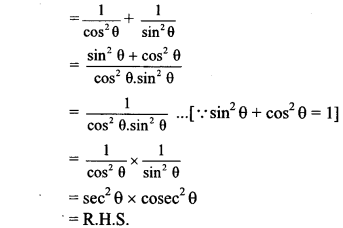∴ sec2 θ + cosec2 θ = sec2 θ × cosec2 θ

iv. L.H.S. = cot2 θ – tan2 θ
= (cosec2 θ – 1) – (sec2 θ – 1)
[∵ tan2 θ = sec2 θ – 1,
cot2 θ = cosec2 θ – 1]
= cosec2 θ – 1 – sec2 θ + 1
cosec2 θ – sec2 θ
= R.H.S.
∴ cot2 θ – tan2 θ = cosec2 θ – sec2 θ

v. L.H.S. = tan4 θ + tan2 θ
= tan2 θ (tan2 θ + 1)
= tan2 θ. sec2 θ
…[∵ 1 + tan2 θ = sec2 θ]
= (sec2 θ – 1) sec2 θ
…[∵ tan2 θ = sec2 θ – 1]
= sec4 θ – sec2 θ
= R.H.S.
∴ tan4 θ + tan2 θ = sec4 θ – sec2 θvii. L.H.S. = sec6 x – tan6 x
= (sec2 x)3 – tan6 x
= (1 + tan2 x)3 – tan6 x …[∵ 1 + tan2 θ = sec2 θ]
= 1 + 3tan2 x + 3(tan2 x)2 + (tan2 x)3 – tan6 x …[∵ (a + b)3 = a3 + 3a2b + 3ab2 + b3]
= 1 + 3 tan2 x (1 + tan2 x) + tan6 x – tan6 x
= 1 + 3 tan2 x sec2 x …[∵ 1 + tan2 θ = sec2 θ]
= R.H.S.
∴ sec3x – tan6x = 1 + 3sec2x.tan2x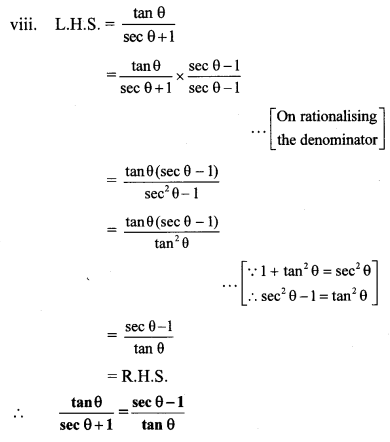x. We know that,
sin2 θ + cos2 θ = 1
∴ 1 – sin2 θ = cos2 θ
∴ (1 – sin θ) (1 + sin θ) = cos θ. cos θ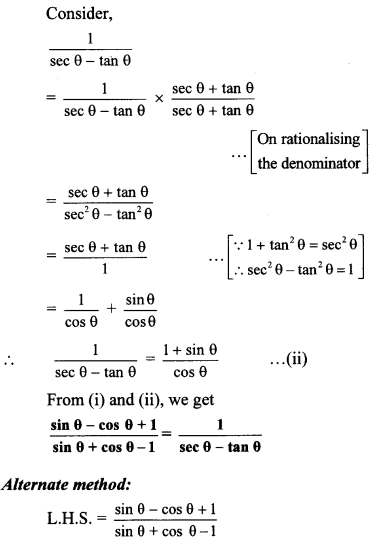Question 6.
A boy standing at a distance of 48 metres from a building observes the top of the building and makes an angle of elevation of 30°. Find the height of the building.
Solution:
Let AB represent the height of the building and point C represent the position of the boy.
Angle of elevation = ∠ACB = 30°
BC = 48 m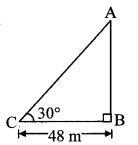In right angled ∆ABC,
tan 30° = $$\frac { AB }{ BC }$$ … [By definition]∴ The height of the building is 16$$\sqrt { 3 }$$ m.

Question 7.
From the top of the lighthouse, an observer looks at a ship and finds the angle of depression to be 30°. If the height of the lighthouse is 100 metres, then find how far the ship is from the lighthouse.
Solution:
Let AB represent the height of lighthouse and point C represent the position of the ship.Angle of depression ∠PAC 30°
AB = 100m.
Now, ray AP || seg BC
∴ ∠ACB = ∠PAC … [Alternate angles]
∴ ∠ACB = 30°
AB = 100m
In right angled ∆ABC,
tan 30° = $$\frac { AB }{ BC }$$ …[By definition]
∴ $$\frac{1}{\sqrt{3}}=\frac{100}{\mathrm{BC}}$$
∴ BC = 100$$\sqrt { 3 }$$m
∴ The ship is 1oo$$\sqrt { 3 }$$m far from the lighthouse.

Question 8.
Two buildings are in front of each other on a road of width 15 metres. From the top of the first building, having a height of 12 metre, the angle of elevation of the top of the second building is 30°. What is the height of the second building?
Solution:
Let AB and CD represent the heights of the two buildings, and BD represent the width of the road.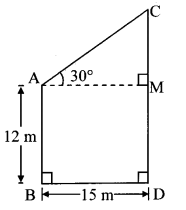Draw seg AM ⊥ seg CD
Angle of elevation = ∠CAM = 30°
AB = 12m
BD = 15m
In ꠸ ABDM,
∠B = ∠D = 90°
∠M 90° …[segAM ⊥ segCD]
∠A 90° …[Remaining angle of ꠸ABDM]
꠸ABDM is a rectangle …[Each angle is 90°]∴ The height of the second building is 20.65 m.

Question 9.
A ladder on the platform of a fire brigade van can be elevated at an angle of 70° to the maximum. The length of the ladder can be extended upto 20 m. If the platform is 2 m above the ground, find the maximum height from the ground upto which the ladder can reach. (sin 70° = 0.94)
Solution:
Let AB represent the length of the ladder and AE represent the height of the platform.Draw seg AC ⊥ seg BD.
Angle of elevation = ∠BAC = 70°
AB = 20 m
AE = 2m
In right angled ∆ABC,
sin 70° = $$\frac { BC }{ AB }$$ …..[By definition]
∴ 0.94 = $$\frac { BC }{ 20 }$$
∴ BC = 0.94 × 20
= 18.80 m
In ꠸ACDE,
∠E = ∠D = 90°
∠C = 90° … [seg AC ⊥ seg BD]
∴ ∠A = 90° … [Remaining angle of ꠸ACDE]
∴ ꠸ACDE is a rectangle. … [Each angle is 90°]
∴ CD = AE = 2 m … [Opposite sides of a rectangle]
Now, BD = BC + CD … [B – C – D]
= 18.80 + 2
= 20.80 m
∴ The maximum height from the ground upto which the ladder can reach is 20.80 metres.

Question 10.
While landing at an airport, a pilot made an angle of depression of 20°. Average speed of the plane was 200 km/hr. The plane reached the ground after 54 seconds. Find the height at which the plane was when it started landing, (sin 20° = 0.342)
Solution:
Let AC represent the initial height and point A represent the initial position of the plane.
Let point B represent the position where plane lands.
Angle of depression = ∠EAB = 20°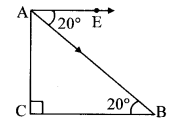Now, seg AE || seg BC
∴ ∠ABC = ∠EAB … [Alternate angles]
∴ ∠ABC = 20°
Speed of the plane = 200 km/hr
= 200 × $$\frac { 1000 }{ 3600 }$$ m/sec
= $$\frac { 500 }{ 9 }$$ m/sec
∴ Distance travelled in 54 sec = speed × time
= $$\frac { 500 }{ 9 }$$ × 54
= 3000 m
∴ AB = 3000 m
In right angled ∆ABC,
sin 20° = $$\frac { AC }{ AB }$$ ….[By definition]
∴ 0.342 = $$\frac { AC }{ 3000 }$$
∴ AC = 0.342 × 3000
= 1026 m
∴ The plane was at a height of 1026 m when it started landing.